Finite Geometry Notes   | Home | Site Map | Author |

Cubist Geometries

by Steven H. Cullinane on Feb. 27, 2010
(revised on Jan. 19, 2011 and on Jan. 13, 2013)

"The cube has…13 axes of symmetry:
6 C2 (axes joining midpoints of opposite edges),
4 C3 (space diagonals), and
3C4 (axes joining opposite face centroids)."
–Wolfram MathWorld article on the cube

These 13 symmetry axes can be used to illustrate the interplay
between Euclidean and Galois geometry in a cubic model
of the 13-point Galois projective plane.

The 13 symmetry axes of the (Euclidean) cube --
exactly one axis for each pair of opposite
subcubes in the (Galois) 3×3×3 cube below --

The geometer's 3 ×3×3 cube --
27 separate subcubes unconnected
by any Rubik-like mechanism --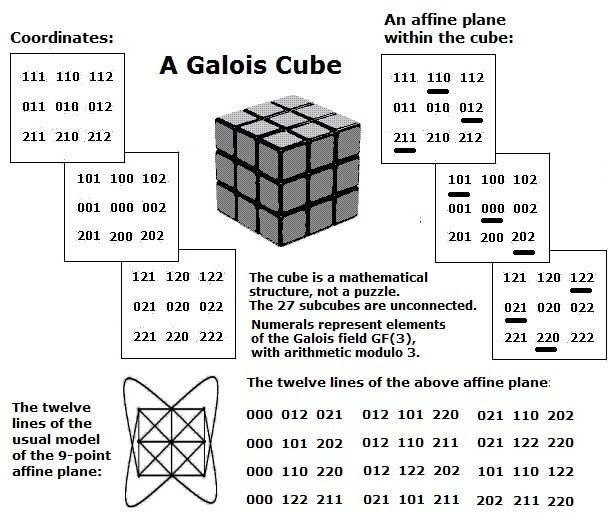A closely related structure --
the finite projective plane
with 13 points and 13 lines --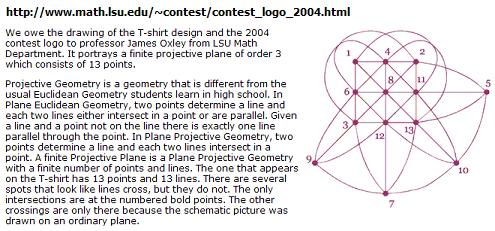The 13 lines of the Galois projective plane
may be derived from 13 Galois affine planes
through the Galois cube's center.

(One such plane is illustrated above.)

These 13 Galois affine planes may in turn be derived from 13
Euclidean planes through the Euclidean cube's center point
perpendicular to the 13 Euclidean axes of symmetry.

A later version of the 13-point plane
by Ed Pegg Jr.–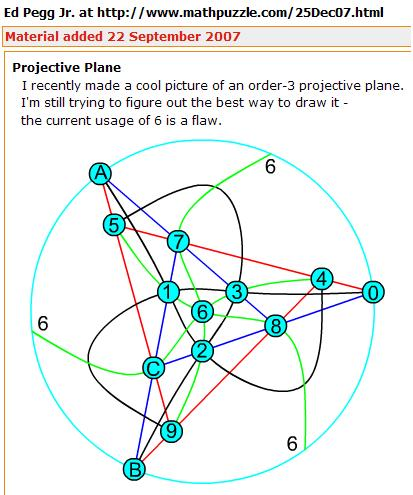A group action on the 3×3×3 cube
as illustrated by a Wolfram program
by Ed Pegg Jr. (undated, but closely
related to a March 26, 1985 note
by Steven H. Cullinane)–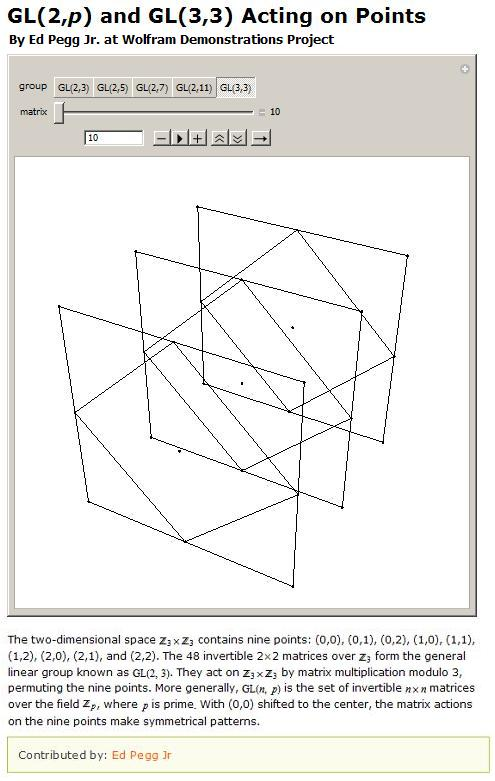at the Mathematical Association of America--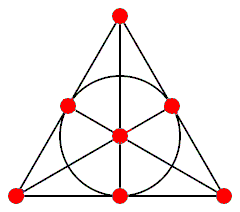The Fano Plane by Ed Pegg Jr., May 30, 2006: “One thing in the Fano plane that bothered me for years (for years, I say) is that it had a circle – and it was described as a line. For me, a line was a straight line, and I didn’t trust curved or wriggly lines. This distrust kept me away from understanding projective planes, designs, and finite geometries for a awhile (for years).”

The above images tell a story of sorts.

The moral of the story --

Galois projective geometries can be viewed
in the context of the larger affine geometries
from which they are derived.

A summary of the story --

The standard definition of points in a Galois projective plane is that they are lines through the (arbitrarily chosen) origin in a corresponding affine 3-space converted to a vector 3-space.

If we choose the origin as the center cube in coordinatizing the 3×3×3 cube (See Weyl's relativity problem), then the cube's 13 axes of symmetry can, if the other 26 cubes have properly (Weyl's "objectively") chosen coordinates, illustrate nicely the 13 projective points derived from the 27 affine points in the cube model.

The 13 lines of the resulting Galois projective plane may be derived from Euclidean planes through the cube's center point perpendicular to the 13 axes of symmetry.

The above standard definition of points in a Galois projective plane may of course also be used in a simpler structure– the eightfold cube.

(The eightfold cube also allows a less standard way to picture projective points that is related to the symmetries of "diamond" patterns formed by group actions on graphic designs.)

Update of January 13, 2013

Summary of how the cube's 13 symmetry planes* are related to
the finite projective plane of order 3, with 13 points and 13 lines--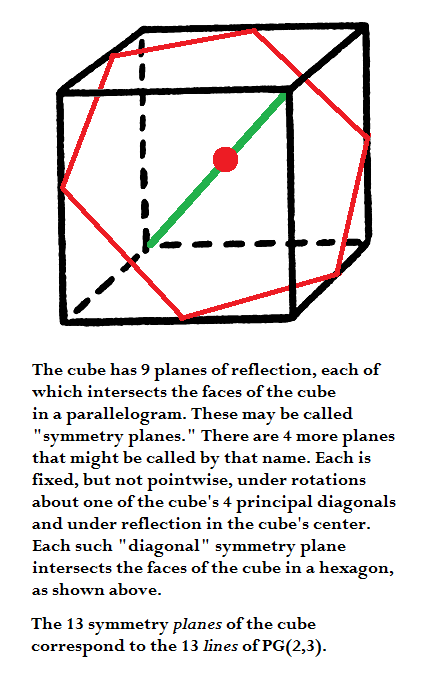* This is not the standard terminology. Most sources count only the 9 planes
fixed pointwise under reflections as "symmetry planes." This of course
obscures the connection with finite geometry.